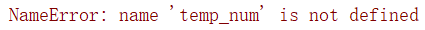# python multiprocessing.pool NameError: name is not defined

Question

The paper code encountered a problem similar to the following. After some efforts, it can be regarded as solving this problem.

``````# import torch
# # print(torch.__version__)
# # print(torch.cuda.is_available())
import multiprocessing
cores = multiprocessing.cpu_count() // 2
def b(xs):

t = temp_num[xs] * 2
return t
def a():
global temp_num
temp_num = [4, 5, 6]
pool = multiprocessing.Pool(cores)
xs = range(3)
batch_result = pool.map(b,xs)
print(batch_result)
def main():
a()
if __name__ == '__main__':
main()
``````

Error:solve

``````import multiprocessing
cores = multiprocessing.cpu_count() // 2
def b(x):
xs = x
temp_num = x
t = temp_num[xs] * 2
return t
def a():
global temp_num
temp_num = [4, 5, 6]
pool = multiprocessing.Pool(cores)
xs = range(3)

z =zip(xs,[temp_num] * 3)
result = pool.map(b,z)
print(result)
def main():
a()

if __name__ == '__main__':

main()
``````